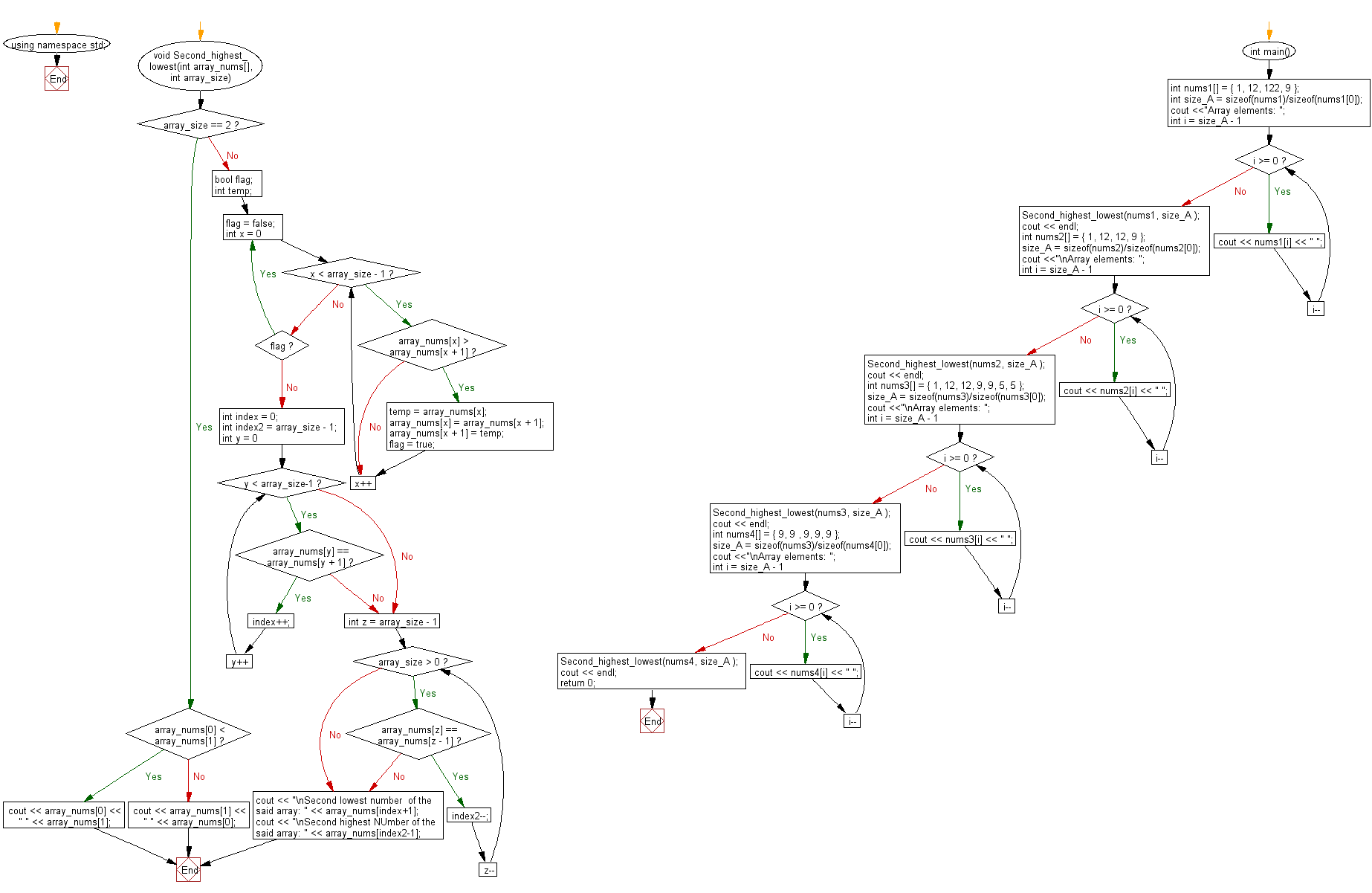﻿ C++: Find the second lowest and highest numbers of an array# C++ Exercises: Find the second lowest and highest numbers in a given array

## C++ Array: Exercise-29 with Solution

Write a C++ program to find the second lowest and highest numbers in a given array.

Sample Solution:

C++ Code :

``````#include <iostream>
#include <string>

using namespace std;

void Second_highest_lowest(int array_nums[], int array_size) {

if (array_size == 2)
{
if (array_nums < array_nums)
{
cout << array_nums << " " << array_nums;
}
else
{
cout << array_nums << " " << array_nums;
}
}
else
{
bool flag;
int temp;

do
{
flag = false;

for (int x = 0; x < array_size - 1; x++)
{
if (array_nums[x] > array_nums[x + 1])
{
temp = array_nums[x];
array_nums[x] = array_nums[x + 1];
array_nums[x + 1] = temp;
flag = true;
}
}
} while (flag);

int index = 0;
int index2 = array_size - 1;
for (int y = 0; y < array_size-1; y++)
{
if (array_nums[y] == array_nums[y + 1])
{
index++;
}
else
{
break;
}
}

for (int z = array_size - 1; array_size > 0; z--)
{
if (array_nums[z] == array_nums[z - 1])
{
index2--;
}
else
{
break;
}
}
cout << "\nSecond lowest number  of the said array: " << array_nums[index+1];
cout << "\nSecond highest NUmber of the said array: " << array_nums[index2-1];
}
}

int main() {

int nums1[] = { 1, 12, 122, 9 };
int size_A = sizeof(nums1)/sizeof(nums1);
cout <<"Array elements: ";
for (int i = size_A - 1; i >= 0; i--)
cout << nums1[i] << " ";
Second_highest_lowest(nums1, size_A );
cout << endl;

int nums2[] = { 1, 12, 12, 9 };
size_A = sizeof(nums2)/sizeof(nums2);
cout <<"\nArray elements: ";
for (int i = size_A - 1; i >= 0; i--)
cout << nums2[i] << " ";
Second_highest_lowest(nums2, size_A );
cout << endl;

int nums3[] = { 1, 12, 12, 9, 9, 5, 5 };
size_A = sizeof(nums3)/sizeof(nums3);
cout <<"\nArray elements: ";
for (int i = size_A - 1; i >= 0; i--)
cout << nums3[i] << " ";
Second_highest_lowest(nums3, size_A );
cout << endl;

int nums4[] = { 9, 9 , 9, 9, 9 };
size_A = sizeof(nums3)/sizeof(nums4);
cout <<"\nArray elements: ";
for (int i = size_A - 1; i >= 0; i--)
cout << nums4[i] << " ";
Second_highest_lowest(nums4, size_A );
cout << endl;

return 0;

}
``````

Sample Output:

```Array elements: 9 122 12 1
Second lowest number  of the said array: 9
Second highest NUmber of the said array: 12

Array elements: 9 12 12 1
Second lowest number  of the said array: 9
Second highest NUmber of the said array: 9

Array elements: 5 5 9 9 12 12 1
Second lowest number  of the said array: 5
Second highest NUmber of the said array: 9

Array elements: 0 0 9 9 9 9 9
Second lowest number  of the said array: 9
Second highest NUmber of the said array: 9
```

Flowchart:C++ Code Editor:

Previous C++ Exercise: Sum up some numbers in an array to get the largest.
Next C++ Exercise: C++ Numbers Exercises Home

--> Next: C++ Numbers Exercises Home

What is the difficulty level of this exercise?

Test your Programming skills with w3resource's quiz.

﻿